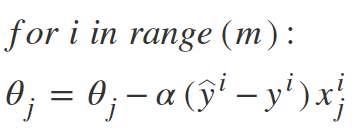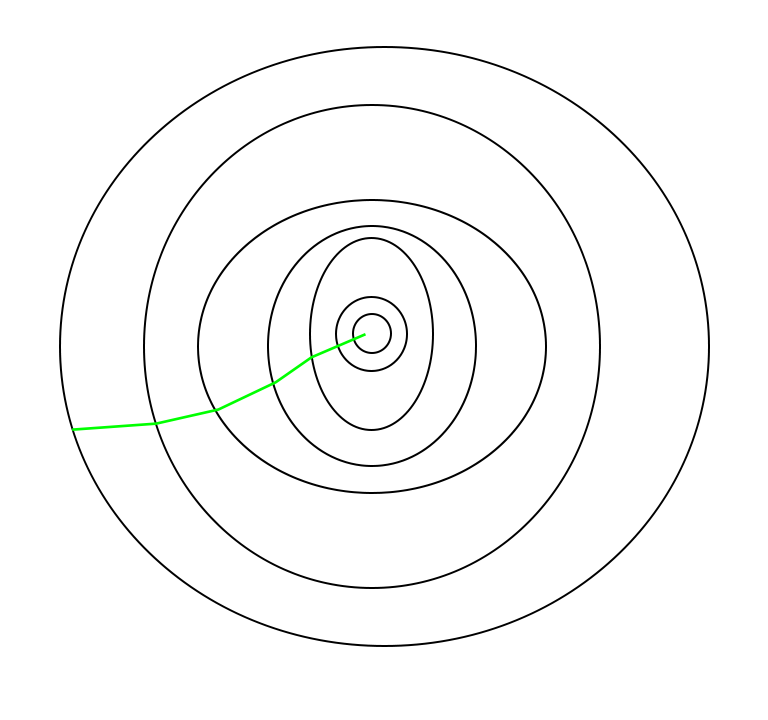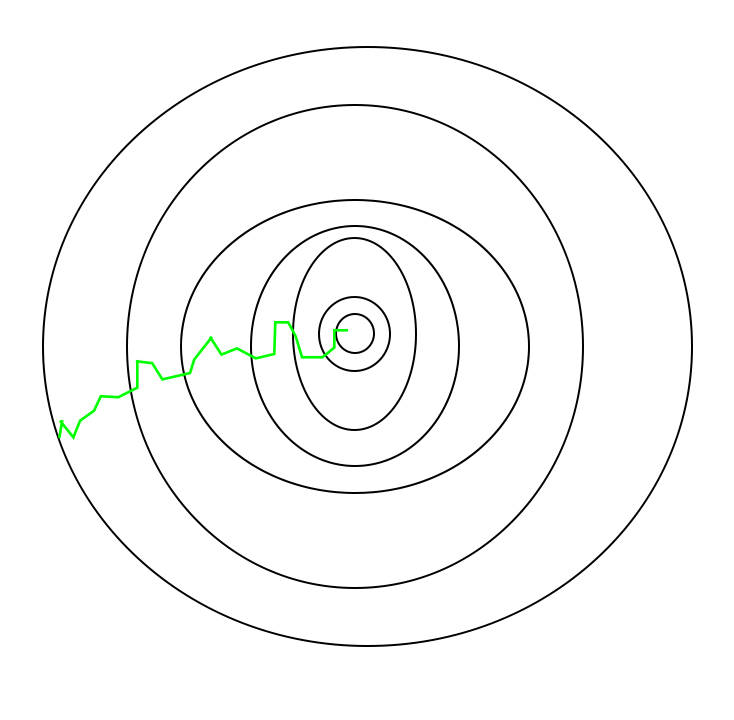# Machine Learning Mastery: Stochastic Gradient Descent (SGD)

Before explaining Stochastic Gradient Descent (SGD), let’s first describe what Gradient Descent is. Gradient Descent is a popular optimization technique in Machine Learning and Deep Learning, and it can be used with most, if not all, of the learning algorithms. A gradient is the slope of a function. It measures the degree of change of a variable in response to the changes of another variable. Mathematically, Gradient Descent is a convex function whose output is the partial derivative of a set of parameters of its inputs. The greater the gradient, the steeper the slope.

Starting from an initial value, Gradient Descent is run iteratively to find the optimal values of the parameters to find the minimum possible value of the given cost function.

Typically, there are three types of Gradient Descent:

The word ‘stochastic‘ means a system or a process that is linked with a random probability. Hence, in Stochastic Gradient Descent, a few samples are selected randomly instead of the whole data set for each iteration. In Gradient Descent, there is a term called “batch” which denotes the total number of samples from a dataset that is used for calculating the gradient for each iteration. In typical Gradient Descent optimization, like Batch Gradient Descent, the batch is taken to be the whole dataset. Although, using the whole dataset is really useful for getting to the minima in a less noisy and less random manner, but the problem arises when our datasets gets big.
Suppose, you have a million samples in your dataset, so if you use a typical Gradient Descent optimization technique, you will have to use all of the one million samples for completing one iteration while performing the Gradient Descent, and it has to be done for every iteration until the minima is reached. Hence, it becomes computationally very expensive to perform.

This problem is solved by Stochastic Gradient Descent. In SGD, it uses only a single sample, i.e., a batch size of one, to perform each iteration. The sample is randomly shuffled and selected for performing the iteration.

SGD algorithm:So, in SGD, we find out the gradient of the cost function of a single example at each iteration instead of the sum of the gradient of the cost function of all the examples.

In SGD, since only one sample from the dataset is chosen at random for each iteration, the path taken by the algorithm to reach the minima is usually noisier than your typical Gradient Descent algorithm. But that doesn’t matter all that much because the path taken by the algorithm does not matter, as long as we reach the minima and with significantly shorter training time.

Path taken by Batch Gradient Descent –Path taken by Stochastic Gradient Descent –One thing to be noted is that, as SGD is generally noisier than typical Gradient Descent, it usually took a higher number of iterations to reach the minima, because of its randomness in its descent. Even though it requires a higher number of iterations to reach the minima than typical Gradient Descent, it is still computationally much less expensive than typical Gradient Descent. Hence, in most scenarios, SGD is preferred over Batch Gradient Descent for optimizing a learning algorithm.

Pseudo code for SGD in Python:

 `def` `SGD(f, theta0, alpha, num_iters): ` `    ``"""  ` `       ``Arguments: ` `       ``f -- the function to optimize, it takes a single argument ` `            ``and yield two outputs, a cost and the gradient ` `            ``with respect to the arguments ` `       ``theta0 -- the initial point to start SGD from ` `       ``num_iters -- total iterations to run SGD for ` `       ``Return: ` `       ``theta -- the parameter value after SGD finishes ` `    ``"""` `    ``start_iter ``=` `0` `    ``theta ``=` `theta0 ` `    ``for` `iter` `in` `xrange``(start_iter ``+` `1``, num_iters ``+` `1``): ` `        ``_, grad ``=` `f(theta) ` `  ` `        ``# there is NO dot product ! return theta ` `        ``theta ``=` `theta ``-` `(alpha ``*` `grad)  `

This cycle of taking the values and adjusting them based on different parameters in order to reduce the loss function is called back-propagation.

# Special 95% discount

## 2000+ Applied Machine Learning & Data Science Recipes

### Portfolio Projects for Aspiring Data Scientists: Tabular Text & Image Data Analytics as well as Time Series Forecasting in Python & R## Two Machine Learning Fields

There are two sides to machine learning:

• Practical Machine Learning:This is about querying databases, cleaning data, writing scripts to transform data and gluing algorithm and libraries together and writing custom code to squeeze reliable answers from data to satisfy difficult and ill defined questions. It’s the mess of reality.
• Theoretical Machine Learning: This is about math and abstraction and idealized scenarios and limits and beauty and informing what is possible. It is a whole lot neater and cleaner and removed from the mess of reality.

Data Science Resources: Data Science Recipes and Applied Machine Learning Recipes

Introduction to Applied Machine Learning & Data Science for Beginners, Business Analysts, Students, Researchers and Freelancers with Python & R Codes @ Western Australian Center for Applied Machine Learning & Data Science (WACAMLDS) !!!

Latest end-to-end Learn by Coding Recipes in Project-Based Learning:

Applied Statistics with R for Beginners and Business Professionals

Data Science and Machine Learning Projects in Python: Tabular Data Analytics

Data Science and Machine Learning Projects in R: Tabular Data Analytics

Python Machine Learning & Data Science Recipes: Learn by Coding

R Machine Learning & Data Science Recipes: Learn by Coding

Comparing Different Machine Learning Algorithms in Python for Classification (FREE)

`Disclaimer: The information and code presented within this recipe/tutorial is only for educational and coaching purposes for beginners and developers. Anyone can practice and apply the recipe/tutorial presented here, but the reader is taking full responsibility for his/her actions. The author (content curator) of this recipe (code / program) has made every effort to ensure the accuracy of the information was correct at time of publication. The author (content curator) does not assume and hereby disclaims any liability to any party for any loss, damage, or disruption caused by errors or omissions, whether such errors or omissions result from accident, negligence, or any other cause. The information presented here could also be found in public knowledge domains.  `